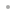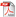Mechanical ExpressionsMechanical ExpressionsFunctionalityExamplesScreencastScreen ShotsDownload Product ManualBuy OnlineRequest a Free Trial# Functionality

Mechanical Expressions provides a wide range of functionality that can be used as the basic building blocks to explore spatial relationships and learn geometric concepts. Mechanical Expressions lets you specify mechanics problems with symbolic constraints rather than entering numeric values. You can also use a combination of numeric and symbolic values for inputs. Request output measurements in symbolic form, as a mathematical expression. Numeric output is also available.

 Mechanical Primitives Point Infinite line Line segment Vector Polygon Circle Ellipse Parabola Hyperbola Arc N-gon Curve approximation Function Piecewise function Constructions Midpoint Intersection Perpendicular bisector Angle bisector Parallel line Perpendicular line Tangent Locus curve Envelope curve Quantitative Constraints Distance, length Radius Angle Direction Slope Coordinates Vector coefficients Proportion along segment or curve Implicit equation Import/Export Send expressions directly to Mathematica MathML import & export Image export: BMP, JPEG, TIFF, PNG, XMP Encapsulated PostScript export Windows Metafile export Scalable Vector Graphics export HTML export Animation (GIF) export HTML5/JavaScript app export Lua app export OS X Dashboard widget export Qualitative Constraints Perpendicular Parallel Incident Tangent Congruent (segments) Transformations Reflection Rotation Dilation Translation Measurements Distance, length Radius Angle Direction Slope Coordinate Area Perimeter Vector coefficients Curve parametric equations Curve implicit equations Mechanics Functionalities Applied Force Applied Torque Mass Moment of Inertia Spring - Damper - Actuator Velocity/Acceleration Reaction force Pin Reaction Resultant Acceleration